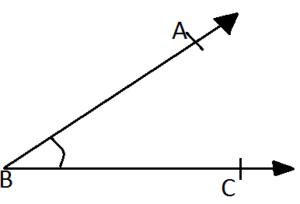# RS Aggarwal Class 6 Math Thirteenth Chapter Angels And Their Measurement Exercise 13A Solution

## EXERCISE 13A

(1) Name three examples of angles from your daily life.

Ans: Scissors, pair of compasses, tongs

(2) Name the vertex and the arms of ∠ABC, given in the figure below.(3) How many angles are formed in each of the figures given below? Name them.(i) Three; ∠BAC,∠ACB and ∠ABC

(4) In the given figure, list the points which(i) are in the interior of ∠AOB = Q, S
(ii) are in the exterior of ∠AOB = P, R
(iii) lie on ∠AOB = A, O, B, T, N

(5) See the adjacent figure and state which of the following statements are true and which are false:(i) Point C is in interior of ∠AOC. = False
(ii) Point C is in interior of ∠AOD. = True
(iii) Point D is in interior of ∠AOC. = False
(iv) Point B is in exterior of ∠AOD. = True
(v) Point C is lies on ∠AOB. = False

(6) In the adjoining figure, write another name for,(i) ∠1 = ∠EPB
(ii) ∠2 = ∠PQC
(iii) ∠3 = ∠FQD

For more exercise solutions, Click Below –Updated: May 30, 2022 — 12:35 pm